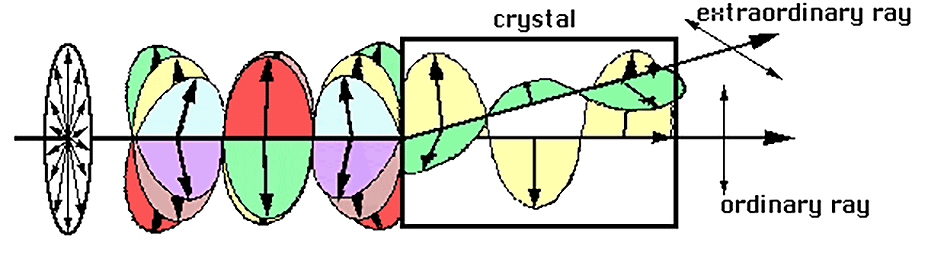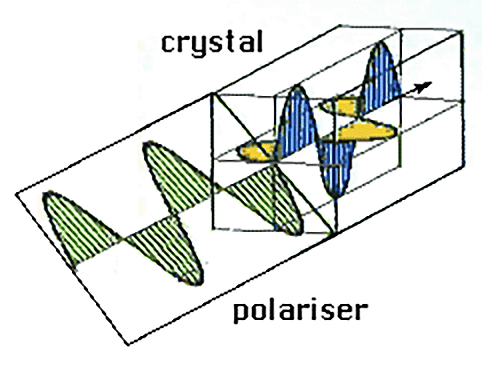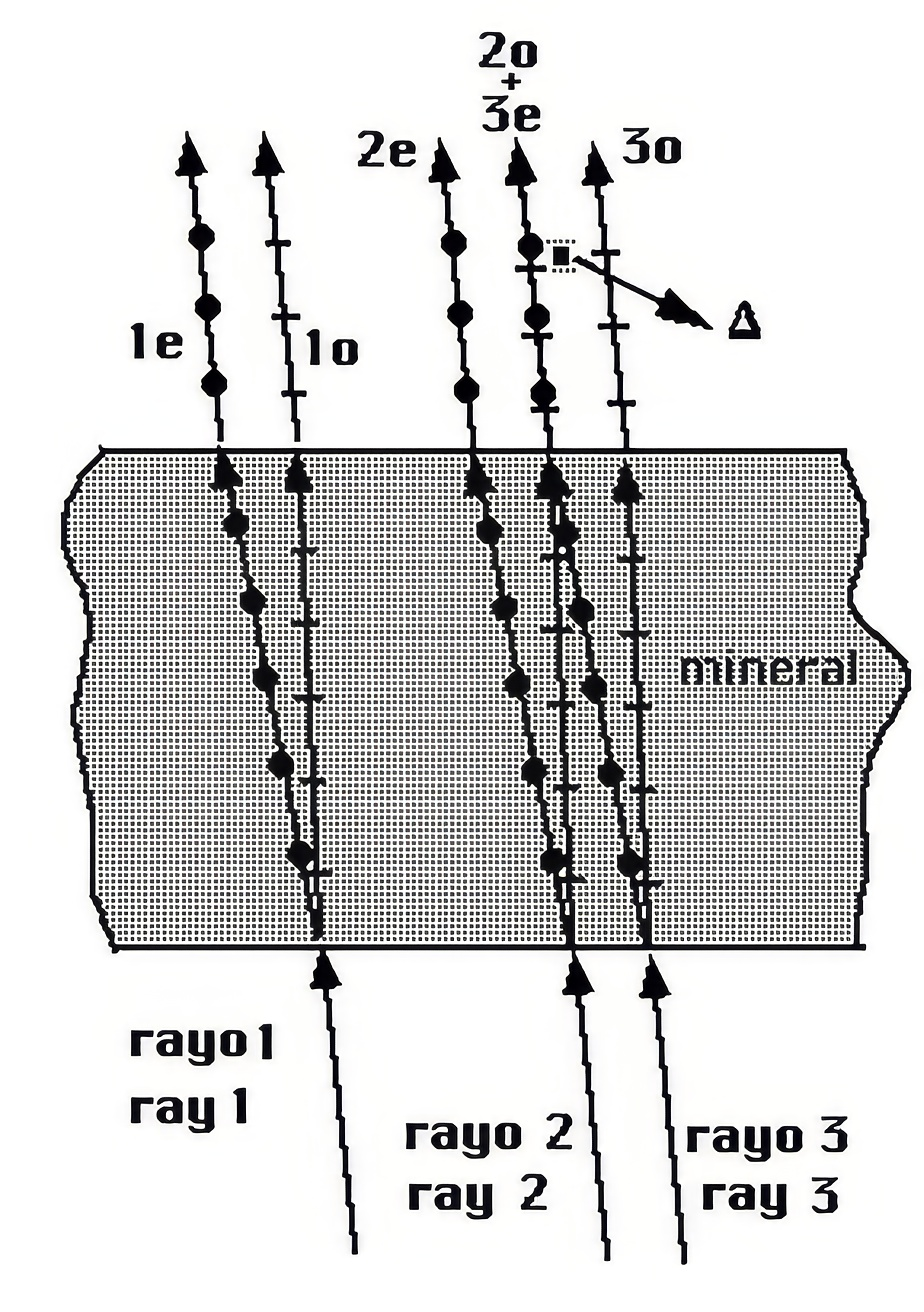Double refraction

Each ray has two waves

When a ray of light arrives at an anisotropic crystal, it splits into two rays of polarised light which vibrate in perpendicular planes.One ray obeys the laws of refraction (ordinary ray) and the other does not (extraordinary ray). Additionally, both present different refractive indices (their directions of vibration are different). When they leave the crystal, both follow parallel paths even though their planes of vibration continue to be perpendicular.

The ordinary and extraordinary component follow different paths inside the crystal, but on leaving the crystal, they follow parallel paths. To simplify matters, we can assume that both components follow a single path although they are vibrating in perpendicular planes.This simplification is correct as, in an emission of light, there are infinite parallel rays and, as is shown in the figure, the extraordinary component of another wave immediately next to it is superimposed by the ordinary component of a wave.

The result is that each wave which arrives at an anisotropic crystal splits into two waves which follow a common path but vibrate in two perpendicular planes.

As the velocity of propagation is determined by the direction of vibration, each wave will vibrate with a different velocity, and on leaving the crystal these will be out of phase ().Index | Introduction | Basic concepts | Previous | Next | Top